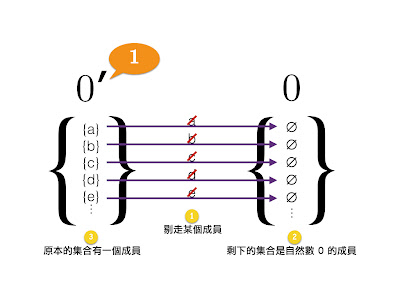12/17/2014 06:59:00 下午Photo Credit: gadl via Compfight cc

(I). $\varnothing\notin\mathbb{N}$

$\{0,1,2,3,4,5,...\}$

$\{0,0^{\prime},0^{\prime\prime},0^{\prime\prime\prime},0^{\prime\prime\prime\prime},0^{\prime\prime\prime\prime\prime},...\}$

「後繼者」是用來刻劃自然數的重要概念。在自然數裡，每個數的後繼者都不一樣。比如， $0$ 的後繼者是 $1$ ， $1$ 的後繼者是 $2$ ， $2$ 的後繼者是 $3$ ，如此類推。所有自然數只有 $0$ 的後繼者是 $1$ ，其他數（如 $1,2,3,4,5,...$）的後繼者都不是 $1$ ；只有 $1$ 的後繼者是 $2$ ，其他數的後繼者都不是 $2$ 。利用後繼者來刻劃，自然數其中一個重要性質是

(A) 沒有兩個不同的自然數有同一個後繼者用符號表達是：$(\forall x)(\forall y)((x\in\mathbb{N}\land y\in\mathbb{N}\land x^{\prime}=y^{\prime})\to x=y)$。

$\{\}, \{a\}, \{b\}, ..., \{a,b\}, \{a,c\}, \{b,c\},... \{a,b,c\}, \{a,c,d\}, ...$

 類型 #2A : $\varnothing$ 類型 #2B : $\{a\}, \{b\}, \{c\}, \{d\}, ...$ 類型 #2C : $\{a,b\}, \{a,c\}, \{b,c\}, \{b,d\}, ...$ 類型 #2D : $\{a,b,c\}, \{b,c,d\}, \{a,b,d\}, \{a,c,d\}, ...$ $\vdots$ $\vdots$

$\{\{a\}, \{b\}, \{c\}, \{d\}, ...\}$

 $0$ = $\{\varnothing\}$ $1$ = $\{\{a\}, \{b\}, \{c\}, \{d\}, ...\}$ $2$ = $\{\{a,b\}, \{a,c\}, \{b,c\}, \{b,d\}, ...\}$ $3$ = $\{\{a,b,c\}, \{b,c,d\}, \{a,b,d\}, \{a,c,d\}, ...\}$ $\vdots$ $\vdots$

$0$ $=_{df}$ $\{\varnothing\}$

$x^{\prime}$ $=_{df}$ 一個集合，這個集合裡的成員 $y$ 都是這樣的：有個 $z$ 在 $y$ 裡面， $z$ 被拿走之後所形成的集合是 $x$ 的成員這個寫法有歧義，其中一個意思是所有 $y$ 都有一個共同成員 $z$ ，但羅素的定義沒有這個意味。 $z$ 只是任何一個 $y$ 裡面任意一個成員，比較嚴格的表達是

$\uparrow\mspace{-6.0mu}y(\exists z(z\in y\land ((y\cap\text{Comp}(\{z\}))\in x)))$

$\uparrow\mspace{-6.0mu}y Fy =_{df} \text{the }w: \forall y(Fy\leftrightarrow y\in w)$
$y\cap w =_{df} \text{the }s: \forall v(v\in s\leftrightarrow (v\in y\land v\in w))$
$\text{Comp}(w) =_{df} \text{the }s: \forall v(v\in s\leftrightarrow v\notin w)$Fig. A: $0^{\prime}$ from $0$ Fig. B: $0^{\prime\prime}$ from $0^{\prime}$

$10 = \{\{a,b,c,d,e,f,g,h,i,j\}\}$
$10^{\prime}=\varnothing$
$10^{\prime\prime}=\varnothing$

Soames, Scott (2005). Philosophical Analysis in the Twentieth Century, Volume 1: The Dawn of Analysis. Princeton University Press.